How Cheenta works to ensure student success?
Explore the Back-Story

# Test of Mathematics Solution Subjective 177 -The Famous Doors ProblemThis is a Test of Mathematics Solution Subjective 177 (from ISI Entrance). The book, Test of Mathematics at 10+2 Level is Published by East West Press. This problem book is indispensable for the preparation of I.S.I. B.Stat and B.Math Entrance.

Also visit: I.S.I. & C.M.I. Entrance Course of Cheenta

## Problem

There are 1000 doors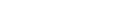and 1000 persons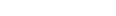. Initially all the doors were closed. Person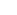goes and opens all doors. Then person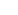closes doorsand leaves all odd numbered doors open. Next,changes the state of every third door, that is,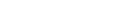by closing a door if it is open or opening a door if it is closed. Similarly,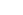changes the state of the doors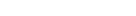, while leaving the other doors untouched. Finally,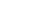opens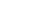if it is closed or closes it if it is open. At the end how many doors remain open?

## Solution

This particular problem requires careful analysis of the problem statements and decide what the problem is exactly asking us to solve.

Basically there are two possible states of the door, namely Open (denote by 'o') and Close (denote by 'c'), and initially all doors are in state 'c'.  Let us define the operation of changing the state of the door by  'F'. So if 'F' can be seen as a function where the variables are 'o' and 'c'  and we have:

F(o) = c

F(c) = o

So if a door is operated on 'n' times we represent it by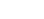as initially all the doors were closed. It is very trivial to state that if the door is operated even number of times it returns to the 'c' state and if it's operated odd number of times then it has a change to 'o' state.

Therefore,= c, if n is even, else= o, if n is odd.       ......(i)

As 'n' represents the number of times the operation has been performed on a particular door, as we understand from the problem that a door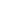is only operated by a personiff k divides m. So whenever we have an divisor of 'm', we increment the value of 'n' by 1. Thus finally after all the counting the value of 'n' will be nothing but the number of divisors of 'm'.

We are to find which doors will remain in state 'o' after the whole procedure and for a door to be in state 'o' it has to be operated odd number of times (from (i)). Which implies that 'n' has to be odd. So all those doors which have odd number of divisors will be open after the process.

Now it can be shown very trivially that a number has odd number of divisors iff it is a perfect square. (Think!!)

So our solution will be all those doors that are marked with perfect square numbers ranging from 1 to 1000.

Solution Set: {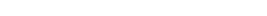}This is a Test of Mathematics Solution Subjective 177 (from ISI Entrance). The book, Test of Mathematics at 10+2 Level is Published by East West Press. This problem book is indispensable for the preparation of I.S.I. B.Stat and B.Math Entrance.

Also visit: I.S.I. & C.M.I. Entrance Course of Cheenta

## Problem

There are 1000 doorsand 1000 persons. Initially all the doors were closed. Persongoes and opens all doors. Then personcloses doorsand leaves all odd numbered doors open. Next,changes the state of every third door, that is,by closing a door if it is open or opening a door if it is closed. Similarly,changes the state of the doors, while leaving the other doors untouched. Finally,opensif it is closed or closes it if it is open. At the end how many doors remain open?

## Solution

This particular problem requires careful analysis of the problem statements and decide what the problem is exactly asking us to solve.

Basically there are two possible states of the door, namely Open (denote by 'o') and Close (denote by 'c'), and initially all doors are in state 'c'.  Let us define the operation of changing the state of the door by  'F'. So if 'F' can be seen as a function where the variables are 'o' and 'c'  and we have:

F(o) = c

F(c) = o

So if a door is operated on 'n' times we represent it byas initially all the doors were closed. It is very trivial to state that if the door is operated even number of times it returns to the 'c' state and if it's operated odd number of times then it has a change to 'o' state.

Therefore,= c, if n is even, else= o, if n is odd.       ......(i)

As 'n' represents the number of times the operation has been performed on a particular door, as we understand from the problem that a dooris only operated by a personiff k divides m. So whenever we have an divisor of 'm', we increment the value of 'n' by 1. Thus finally after all the counting the value of 'n' will be nothing but the number of divisors of 'm'.

We are to find which doors will remain in state 'o' after the whole procedure and for a door to be in state 'o' it has to be operated odd number of times (from (i)). Which implies that 'n' has to be odd. So all those doors which have odd number of divisors will be open after the process.

Now it can be shown very trivially that a number has odd number of divisors iff it is a perfect square. (Think!!)

So our solution will be all those doors that are marked with perfect square numbers ranging from 1 to 1000.

Solution Set: {}

### Knowledge Partner